# Relative Speed

“Relative” means “in comparison to”. Thus, the concept of relative speed is used when two or more bodies moving with some speeds are considered. To make things simpler, one body can be made stationary (i.e. Speed =zero) and take the speed of the other body with respect to the stationary body, which is the sum of the speeds if the bodies are moving in the opposite direction and the difference if they are moving in the same direction. This speed of the moving body with respect to the stationary body is called the relative Speed.

Relative Speed of 2 bodies = Sum of their individual speeds if they are moving in the opposite direction = Difference of their individual speeds if they are moving in the same direction.

Consider A and B starting at the same time, travelling in the opposite direction with a speed of 20 kmph and 30 kmph and they have to travel a distance of 200 km. Now the concept of relative speed can be used to find the time of their meeting.

Two bodies moving towards each other are A and B with speeds 20 kmph and 30 kmph. Now make body A stationary and take the speed of B in reference to A, so relative Speed of B = sum of their respective speeds = 20 + 30 = 50 kmph ( as they are travelling in opposite directions).

Now A can be assumed to be stationary and the total distance has to be travelled by B which he will do with a Speed = 50 kmph. So he will meet A after 200/50 = 4 hrs.

You may also want to check out these topics given below!Relative speed is one of the most interesting topics which is central to most physics concepts. When do you say that an object is in motion? If an object is lying completely still on the ground, is it in motion or at rest? To understand these questions one must learn the relative speed concept.

## What is Relative Speed?

We can define relative speed as the speed of a moving body with respect to another. When two bodies are moving in the same direction, the relative speed is computed by their difference. But when to bodies are moving in the opposite direction, the relative speed is calculated by adding the speed of both the bodies.

The difference between relative speed and relative velocity is that relative speed is the scalar quantity whereas relative velocity is the vector quantity.

### How to calculate relative speed?

Suppose two bodies are moving at a different speed in the same direction.

Let the speed of 1st body be x km/hr
And the speed of the 2nd body be y km/hr.

So, their relative speed is = (x – y) km/hr [x > y]

Then,

The time after which both the bodies meet = distance travelled / relative speed

= d km/ (x – y) km/hr

Now, we already know that relative speed is the speed of one object with respect to another.

So, when time after which both the bodies is given,

Suppose, time = t hrs.

Then, the distance covered in ‘t’ hours = relative speed * time

= (x – y) km/hr * t hrs.

### Relative Speed Examples

Example 1:

The thief Bhagu Ram is spotted by the policeman Pakad Singh from a distance of 200m. Once they see each other they start running. What is the Distance Bhagu Ram who is running at 5 kmph would have covered before being caught by Pakad Singh running at 7 kmph?

Solution:

Here are two bodies moving at different Speeds. So assume Bhagu Ram to be stationary and take the Speed of Pakad Singh relative to Bhagu Ram. So, now once the concept of relative Speed is applied, it can be deduced that Bhagu Ram is standing and Pakad Singh who is at a distance of 200 m is running at 7 – 5 = 2 kmph. To catch Bhagu Ram he needs to travel a distance of 200 ms or 0.2 km. So Time taken = 0.2/2 = 0.1 hours or 6 minutes. Thus the Distance that is covered by Bhagu Ram before being caught is 0.5 km.

Example 2:

A bird is sitting on a train A moving at a speed of 40 kmph. It sees another train B at a Distance of 200 ms with Speed of 60 kmph coming from the opposite direction on the same rail track. It flies with an average speed of 10kmph and sits on another train. Again immediately it flies back to the first train and again to the second train and so on. It does so before the two trains crash. What is the total distance travelled by the bird?

Solution:

Two trains are moving in opposite direction with Speed 40 kmph and 60 kmph. Make train B stationary and then take the Speed of train A relative to the train B. So relative Speed of train A = 40 + 60 = 100 kmph. Before it crashes into the stationary train B it has to travel a Distance of 200 m which it will do in (0.2/100 ) hours. During this duration, the average Speed of bird has been 10 kmph i.e. before the crash total Distance covered by the bird = 0.2 * 10/100 km = 20 meters.

### Some Specific Cases of Relative Speed

#### CASE 1 : Boats & Streams

Let the Speed of boat in still water = x

Speed of stream = y

As it is previously discussed that in questions where two bodies with different Speeds are concerned, the concept of relative speed should be used. Here the stream can be assumed to be the stationary body and the Speed of boat relative to the Speed of the stream can be taken. Using the concept of relative Speed, one can now have the situation where the stream is stationary and only the boat is moving at a speed determined by the direction of the boat relative to the stream.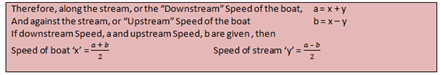Example 3:

Gita rows a boat at a speed of 15 kmph upstream and 20 kmph downstream. Find the Speed with which Gita rows the boat in still water and also find the Speed of the stream.

Solution:
Given that upstream Speed = 15 kmph

Downstream Speed = 20 kmph

Speed of Gita in still water = x = ((a + b))/2= (20+15)/2 = 35/2

Speed of stream =y = ((a – b))/2= (20-15)/2 = 5/2.

Example 4:

The Speed of Narmada river is 5 kmph. A stationary body is placed in the river. Find the Time taken by the floating body to reach a stone which is 10 km downstream from the point where it is now?

Solution:

Speed of body = Speed of river (as Speed of boy is 0) = 5 kmph

Speed=Distance/Time.

So, Time taken to reach 10 km = 10/5 = 2 hours.

Example 5:

A man rows 135 km upstream in 2.7 hours. He rows the same distance downstream in 2.5 hours. However, for downstream, he reduces his Speed by 9%, while Speed of the current gets reduced by 20%. Find the Speed of the man.

(a) 52

(b) 55

(c) 50

(d) none of these

Solution:

Let us use a different approach for this question

x= The speed of boat and

y= The Speed of stream

Given that x-y= 135/2.7= 50 —–(1) & Given that 10/11 x + 4/5 y = 135/2.5 = 54 ——–(2)

(reduction of 9% = 100- 9 = 0.91x. 0.91 in terms of a fraction can be written as 10/11 since 1/11= 0.0909). (Similarly, reduction of 20% => 0.8 y. 0.8 in terms of a fraction can be written as 4/5.

Option (c)The Speed of the man cannot be 50, as the Speed of the stream cannot be zero (on substituting in equation (1))

option (b) substitute x= 52 and x=55 in the second equation and see for which value you are getting an integral value for y. This happens only for x=55, where you will get y=5. Hence answer is the option (b) Here the Reverse Gear approach is used i.e. working from answer options. It should be Remembered that longer the question, the easier it is to eliminate the wrong answer options.

#### CASE 2: Moving body and a stationary body of negligible length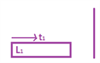Assume a stationary pole and a train approaching it. Here the Speed of the train relative to the pole will be the Speed of the train itself, as the pole has a Speed of zero. Also, since the pole is of negligible length in comparison to the train, the Distance travelled by train to pass the pole will be the length of the train itself. Therefore, the Time taken by a train with length L1 and Speed S1 to pass a post/ stationary pole = L1/S1

Example 6:

A train is travelling at a speed of 4 m/s. Raman is standing on the road. How much time will it take for the train to cross him if the length of the train is 200m?

Solution:

Time taken to pass Raman= (Length of train)/(Speed of train) = 200/4= 50 sec.

#### CASE 3: Moving body and a stationary body of some length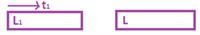Now let’s assume that the same train is going to pass a long bridge of length L. The new Distance covered by the train while passing over the bridge will be the length of the bridge+ the length of the train. Also, the Speed of the train relative to the bridge will be the Speed of the train itself, as the bridge is stationary. The time taken by a train of length L1 and Speed S1 to pass a bridge of length L = (L1+ L)/S1 Here the Distance to be covered is the sum of the lengths of train and bridge.

#### CASE 4: Two moving bodies in opposite directions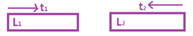When two bodies move in opposite direction, then the Relative Speed = Sum of Speeds i.e for e.g. for a person sitting in a train moving with a Speed of 40 km/hr in the west direction, another train which is going towards east with a Speed of 40 km/hr, will appear to move at a Speed of (40+40) = 80 km/hr. There are two trains with lengths L1 and L2 and Speeds S1 and S2 respectively moving in the opposite direction. Time taken by the trains to cross each other after meeting = (L1+L2)/(S1+ S2 ).

Here the Total Distance to be covered is the sum of the lengths of the trains and relative Speed is the sum of the Speeds (since they are moving in the opposite direction).

#### CASE 5: Two moving bodies in the same direction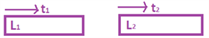When two bodies move in the same direction then the Relative Speed = Difference of Speeds i.e for a person a sitting in a train moving with a speed of 60 km/hr in the west direction, another train which is going towards the west with a speed of 40 km/hr, will appear to move at a Speed of (60-40) = 20 km/hr.

There are two trains of lengths L1 and L2 and traveling at Speeds S1 and S2 respectively moving in the same direction. Time taken by the trains to cross each other after meeting = (L1+L2)/(S1 – S2 ).

Here the Total Distance to be covered is the sum of the lengths of the trains and relative Speed is the difference of the Speeds (since they are moving in the opposite direction).

Example 7:

Train A which is 125 m long is travelling at 108 km/hr. On a parallel track, a 180 m long Train B is travelling at 72 km/hr, in the same direction. In Train B, a passenger is walking towards the rear end of the train at a speed of 9 km/hr. In how many seconds will Train A completely cross the passenger in Train B?

(a) 14.4

(b) 10

(c) 16.67

(d) 24

Solution:

Speed of A = 108 km/hr = 30 m/s

Speed of train B = 72 km/hr = 20 m/s

Here r the relative Speed between train A and train B should be considered first i.e.= 30 -20 = 10 m/s.

Relative Speed -Train A and man moving in the opposite direction with a Speed of 2.5 m/s=10 +2.5 = 12.5m/s

Time taken by train A to pass the man who is moving in the opposite direction = 125/12.5 = 10 secs since the Distance to be covered over here is the length of train A only.

Example 8: How much Time a 100m long train travelling at a speed of 8 m/s will take to overtake another train which is 80m and is travelling in the same direction at a speed of 4 m/s?

Solution:

Suppose the 80 m long train is stationary and take the Speed of the 100m long train in relative to the former. So relative Speed of 100m long train = 8 – 4 = 4 m/s. To overtake the 80m long train it has to travel a total Distance = sum of its own length and that of the other train = 100 m + 80 m = 180 m So to travel that Distance with a Speed of 4 m/s, it will take 180/4= 45 secs.

### Solved Example of Relative Speed

Question:Two vehicles are travelling from the same location at the speed of 6 km/hr and 4 km/hr respectively. Calculate the distance between the vehicles after 10 minutes given that both vehicles are travelling in the same direction.

Solution:The relative speed of the vehicles when they move in the same direction

= (6 – 4) km/hr

= 2 km/hr

Total time taken = 10 minutes

Therefore distance travelled = speed × time
= (2 × 10/60) km
= 1/3 km
= 1/3 × 1000 m
= 333.3 m

Therefore, the distance between the vehicles after 10 minutes is 333.3 m given that both vehicles are travelling in the same direction.Stay tuned with BYJU’S to learn more interesting topics and related concepts like relative velocity, relative speed formula, etc. with the help of interactive video lessons.

Test your Knowledge on Relative Speed

#### 1 Comment

1. This is very helpful for me so thankyou Byjus